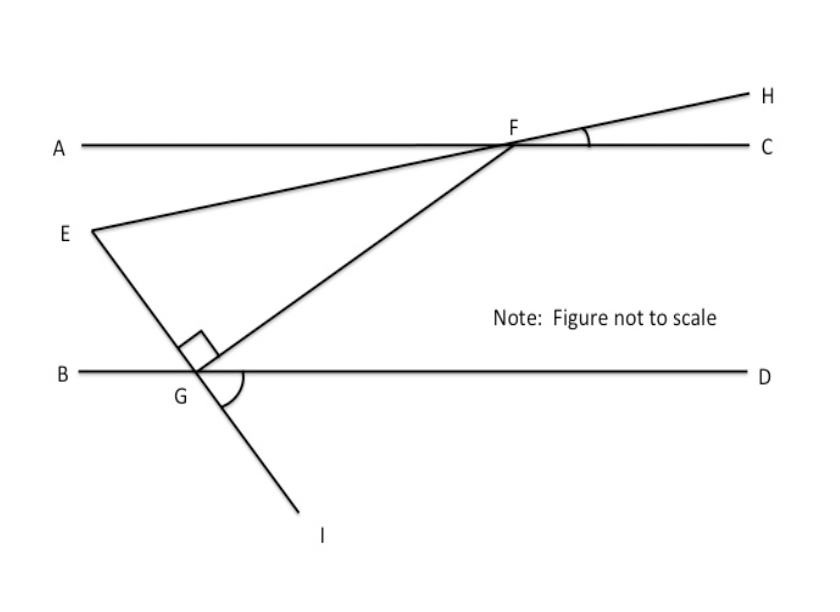# ACT Mathematics: Coordinate Geometry and Trigonometry Quiz

This is an MCQ-based quiz on the topic of ACT Mathematics: Coordinate Geometry and Trigonometry Quiz.

This set of MCQs helps you brush up on the important mathematics topic and prepare you to dive into skill practice and expand your knowledge to tackle the question and answer it carefully.

Start Quiz

Lines AC and BD are parallel.  ∠HFC=10∘, ∠DGI=50∘, △EFG is a right triangle, and  EG has a length of 10.  What is the length of  EF?20

5

15

12

One-half of the measure of the supplement of angle ABC is equal to the twice the measure of angle ABC. What is the measure, in degrees, of the complement of angle ABC?

72

45

54

18

Two angles are supplementary and have a ratio of 1:4.  What is the size of the smaller angle?

45

18

144

36

Solve the following equation:

(9 + 1) * (4^2 + 2) * (7^2 + 1) / 2 = ?

39

9000

4500

1500

{10, 12, 24, 50, 60, 100, 260, 480, 606, 1000}

What is the probability that a number selected randomly from the set will be divisible by both 4 and 6?

1/2

2/5

3/10

1/10

If x percent of 300 is 120, what is 175% of x?

310

70

50

320

Two dice are rolled. What is the probability of getting two odd numbers on two dice?

1.6

2.3

1/2

1/4

Circle A is centered about the origin and has a radius of 5. What is the equation of the line that is tangent to Circle A at the point (–3,4)?

3x – 4y = –25

3x – 4y = 25

3x + 4y = –25

-3x + 4y = –29

Give the equation, in slope-intercept form, of the line tangent to the circle of the equation

x^2+y^2=225

at the point (12,−9).

y = (4/3)x−25

y = -(4/3)x−25

y = (4/6)x−25

y = (4/3)x−20

Give the equation, in slope-intercept form, of the line tangent to the circle of the equation

x^2−6x+y^2−91=0

at the point (−3,8).

y = 3/4x + 41/4

y = 3/4x + 41/6

y = -3/4x + 41/4

y = 3/4x - 41/4

Quiz/Test Summary
Title: ACT Mathematics: Coordinate Geometry and Trigonometry Quiz
Questions: 10
Contributed by: